## Want to keep learning?

This content is taken from the Raspberry Pi Foundation & National Centre for Computing Education's online course, Programming 102: Think Like a Computer Scientist. Join the course to learn more.
3.3

## Raspberry Pi Foundation

Skip to 0 minutes and 3 seconds In this step, we’re going to look at a method of sorting lists called the Bubble Sort. The Bubble Sort algorithm is one of the simplest sorting algorithms to implement. However, it’s poor efficiency means that it’s more often used as a teaching tool to introduce the concept of sorting rather than an algorithm that’s actually used in practise. This means that virtually every student of computer science will, at some point or another, learn how the Bubble Sort works. Let’s look at the Bubble Sort algorithm in the real world. Imagine there are five cars, all travelling down a straight road. The cars are all being driven on cruise control but each at a different speed.

Skip to 0 minutes and 42 seconds When a car is travelling faster than the car in front, it will overtake it and occupy the slower car’s position in the traffic. This will keep occurring, with the cars switching positions with any slower car in front of them. Eventually, the cars will sort themselves according to their speeds, with the fastest car being at the front of the queue and the slowest at the back. This is similar to how the Bubble Sort algorithm works. Let’s take a look.

Skip to 1 minute and 10 seconds In the following example, we’ll use the Bubble Sort to organise a variety of balls used in some different sports, according to their size. We’ll start with the balls in a random order, lined up in a horizontal line. We’ll use a variable called Swapped to track whether we’ve swapped any balls as we go. As yet, we’ve not changed any balls around. So we’ll set Swapped to False. Then we compare the first two balls in the line.

Skip to 1 minute and 39 seconds We can see that the tennis ball is smaller than the soccer ball, so there’s no need to swap their positions. Our swap variable remains false. Next, we compare the soccer ball with the pool ball. The soccer ball is larger than the pool ball, so we need to swap their positions.

Skip to 2 minutes and 1 second Because we’ve made this change, we’ll also need to make sure that Swapped is set to True. Next, we compare the soccer ball with the basketball. They’re the right way around. We don’t need to swap them. We keep progressing down the line, swapping balls if needed.

Skip to 2 minutes and 21 seconds Once we’re at the end, we can check the Swapped flag. If it’s true, we need to go back and look at all the balls again.

Skip to 2 minutes and 41 seconds As you can see, repeated repetitions of the algorithm result in the balls all being sorted. Eventually, once all the balls are in order, the arm will go down the row, measure each ball, but not need to swap any. At this point, Swapped remains False. And the loop can come to an end.

Skip to 3 minutes and 5 seconds So to summarise, the Bubble Sort algorithm begins by setting Swap to False, checks each pair of items in the set comparing their size. If the one on the left is greater than the one on the right, the balls are swapped. If not, they’re ignored. At the end of the set, if a swap has occurred, Swapped is reset to False. And the set is examined once again. Once the robot arm gets to the end without making any swaps, then it knows that the set is sorted. In the next step, we’ll look at implementing this in Python.

# An introduction to Bubble Sorts

In this step, we’re going to look at a method of sorting lists called bubble sort.

The bubble sort algorithm is one of the simplest sorting algorithms to implement. It’s not a very widely used sorting algorithm, but is more often used as a teaching tool to introduce the concept of sorting. This means that virtually every student of computer science will, at some point or another, learn how bubble sort works.

In this step we’ll take a broad look at the bubble sort algorithm, try to explain how it works, and provide a visualisation of the algorithm in action. Later on you’ll learn why it is not the best algorithm to sort a million 32-bit integers.

Real-world examples of the bubble sort algorithm are hard to find. However with a little imagination, we can see how a bubble sort might happen in a real situation.

Imagine there are five cars all travelling down a straight road. They are all being driven on cruise control, but each of the cars’ speeds have been set to slightly different values.

When a car is travelling faster than the car in front, it will overtake it, and occupy the slower car’s position in the traffic. This will keep happening, with each car switching positions with any slower car in front of it. Eventually the cars will sort themselves according to their speeds, with the fastest car being at the front of the queue of traffic.

This is in essence how bubble sort works.

Let’s look in a little more detail at what is going on with the algorithm when we use it to sort some data. In the following example, we will sort some different balls, used in a variety of sports, according to their size. We’ll start with the balls in a random order, lined up in a horizontal line.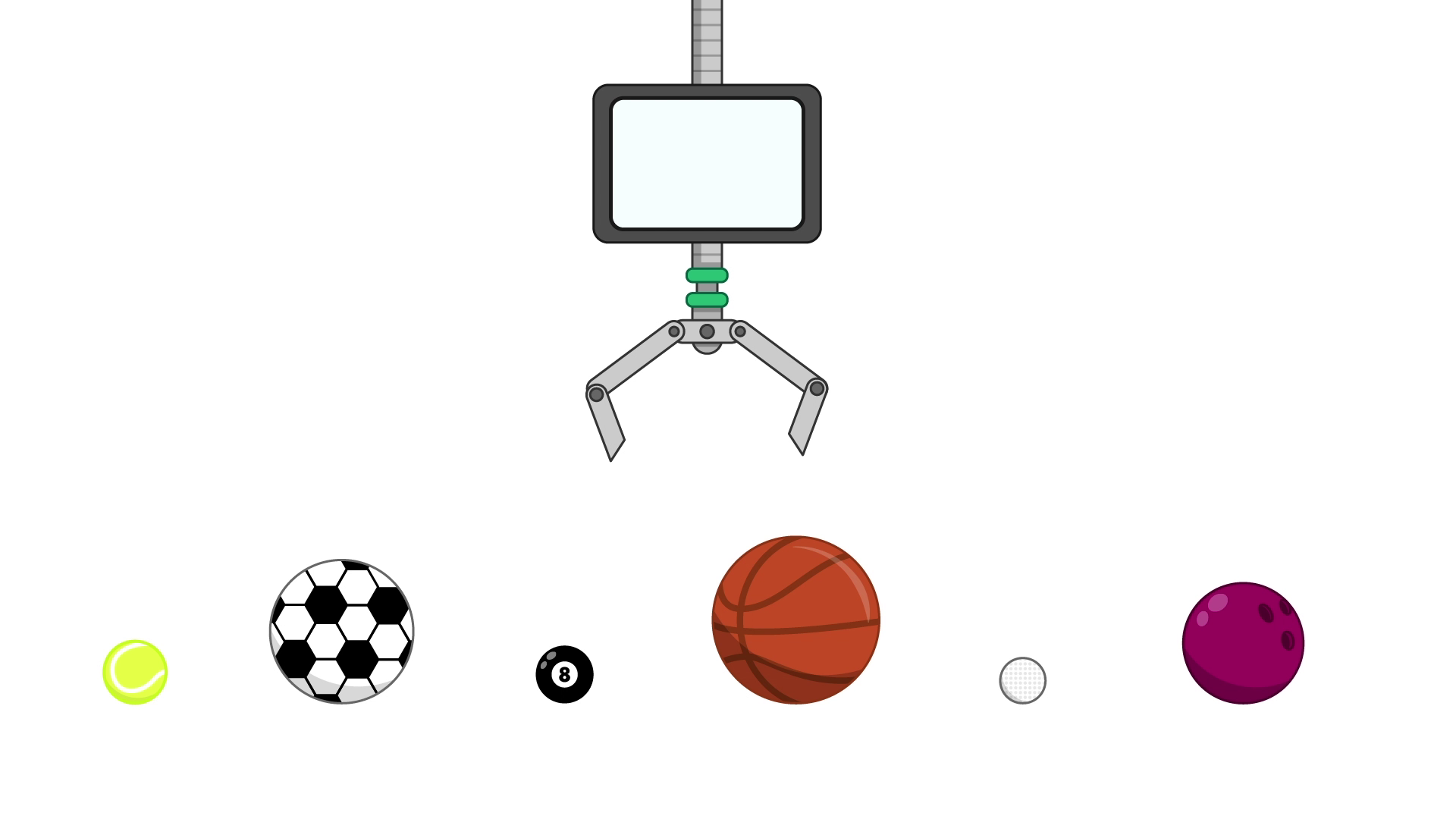1. We can start by setting a variable that states we’ve changed the order of the list. As the list is jumbled (or might be), we set swapped = True.
2. Now, we want to keep repeating our sorting actions, until we find we haven’t made any changes to the order of the balls since the last time through. This is because if we’ve looked at all the balls’ positions and have made no changes, the balls must be sorted.
3. As we’ve yet to change the order of any balls, we’ll set swapped to False. Then we can compare the first two balls in the line.
4. We can see that the tennis ball is smaller than the soccer ball, so there is no need to change their positions.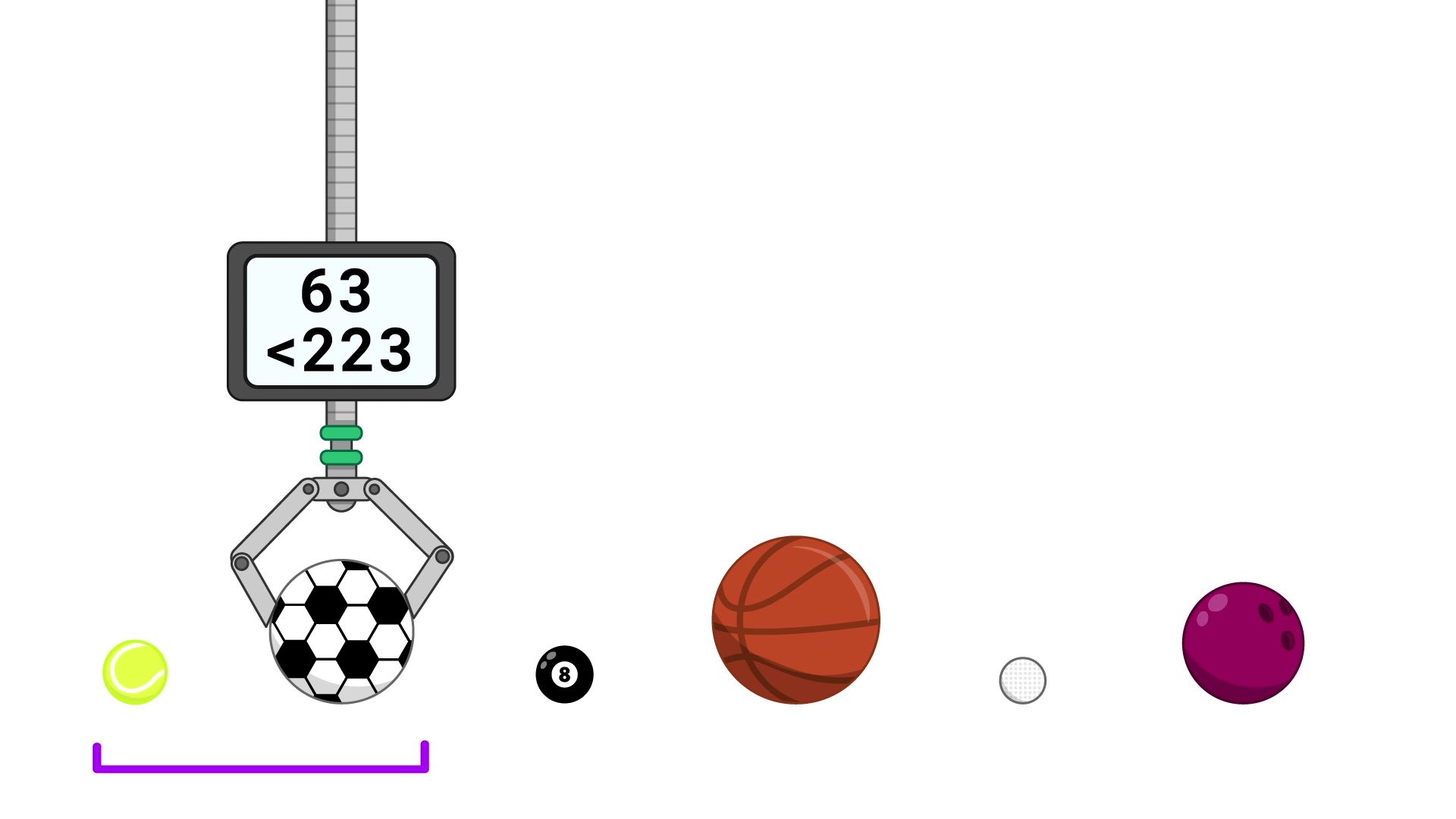5. swapped remains False as no changes to the order have been made.
6. Next we compare the soccer ball with the pool ball. The soccer ball is larger than the pool ball, so we can swap their positions.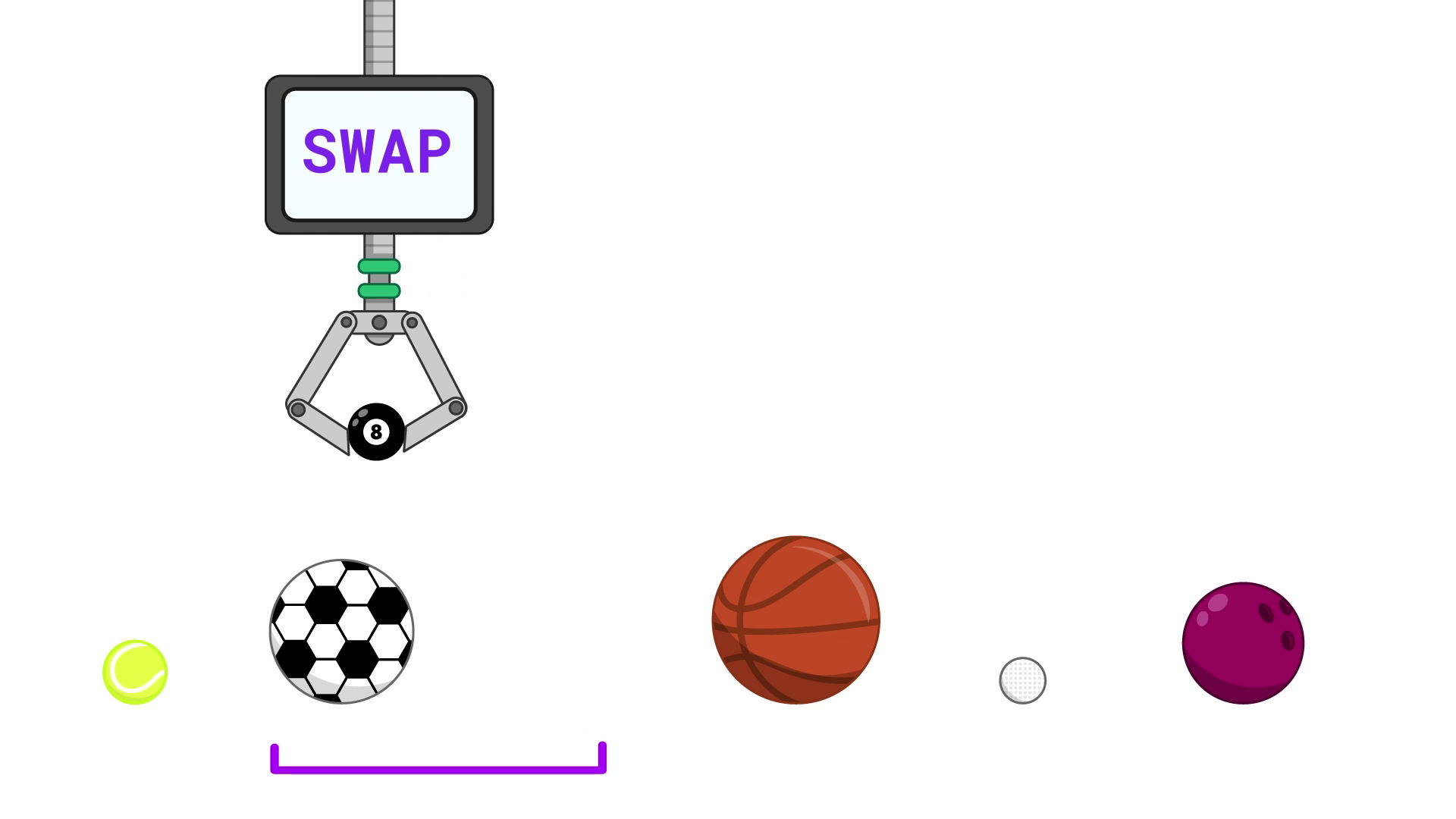7. Because we’ve made a change to the order of the balls, we need to make sure that swapped = True.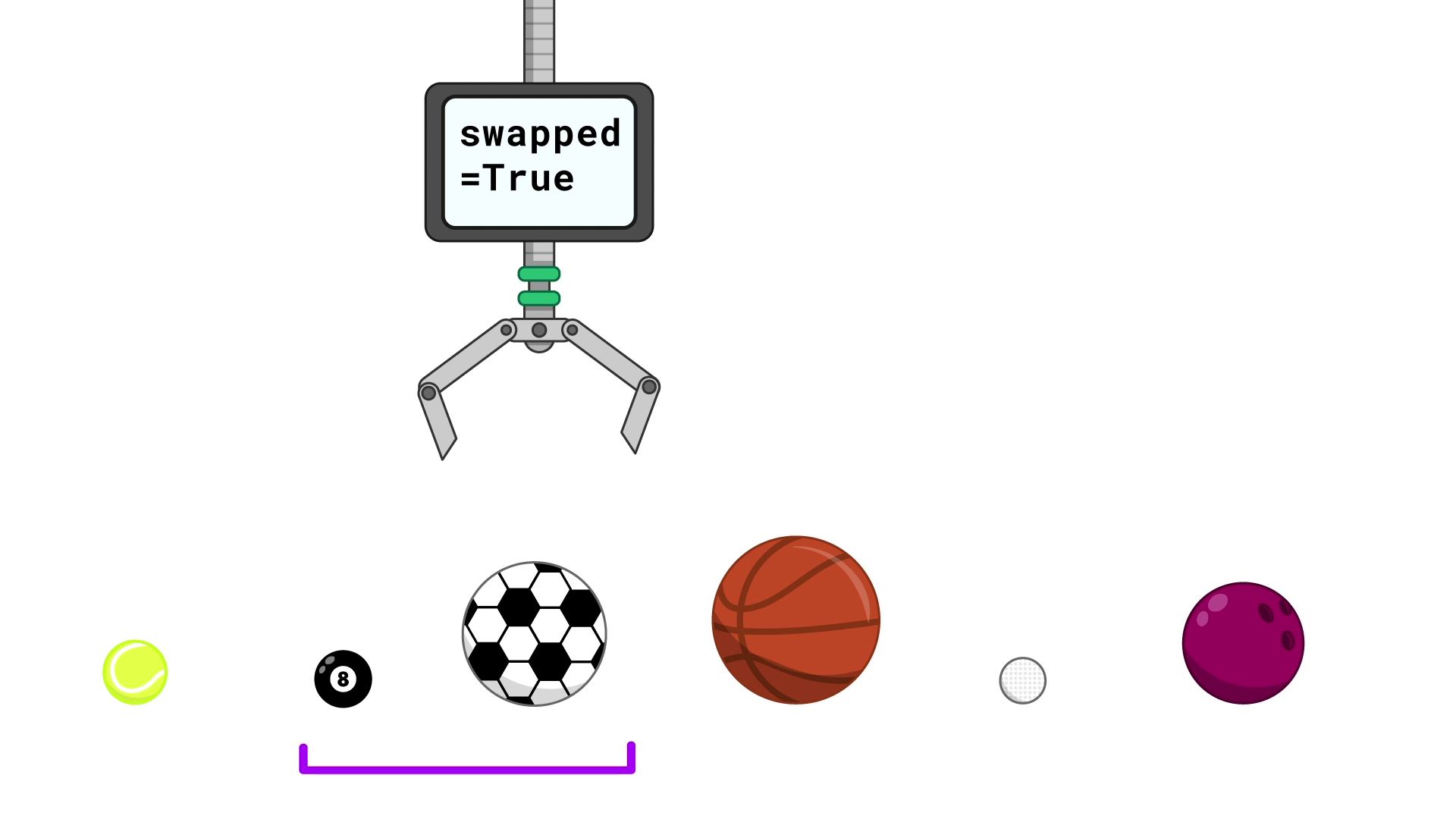8. Now we compare the soccer ball with the basketball. The basketball is larger, so again, we don’t need to swap them.
9. We keep progressing down the line, swapping the balls if needed.
10. Once we’re at the end, we can check the swapped flag. As we have made a change to the order, we will find it is True, so we need to go back to step 2.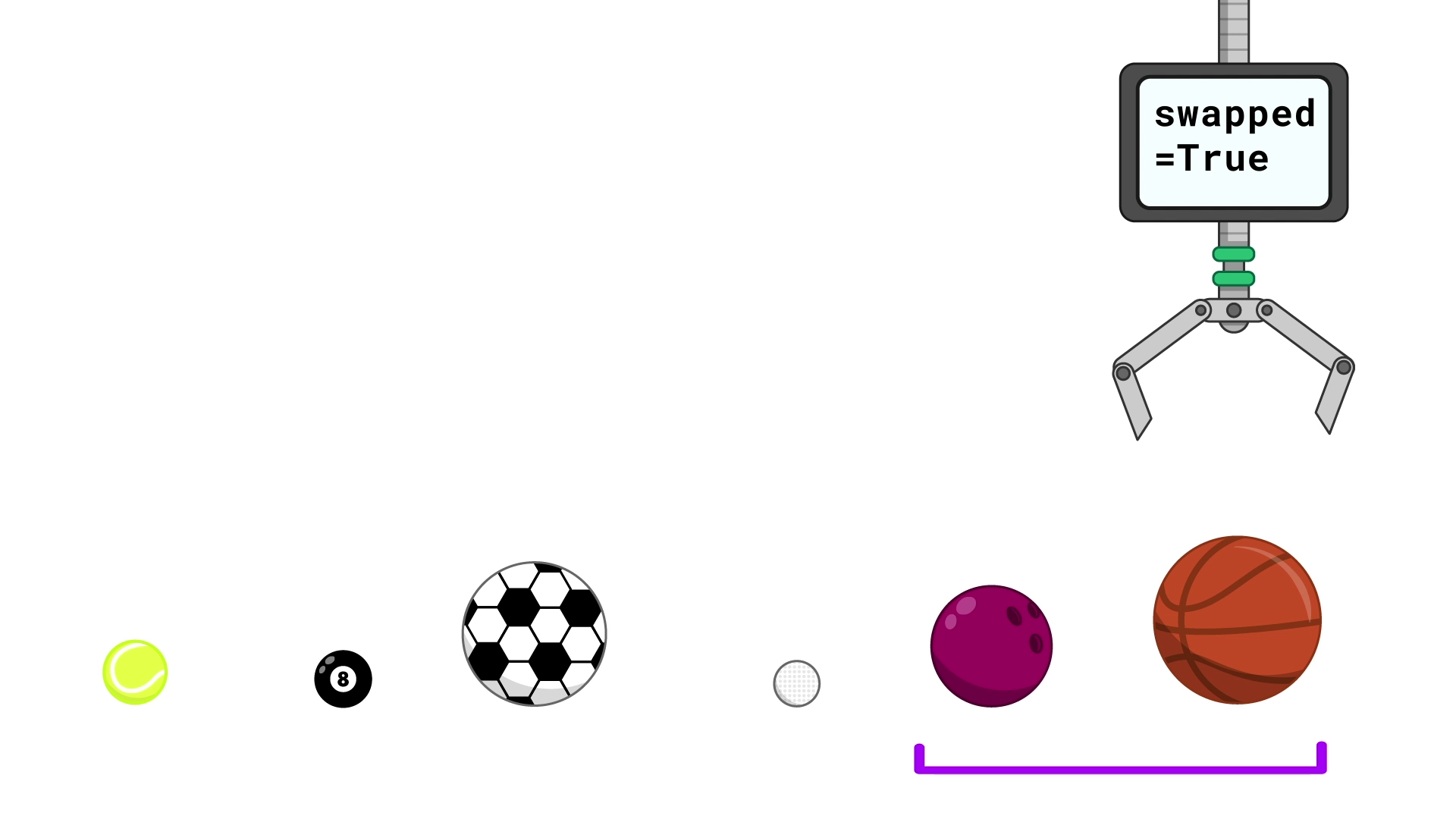As you can see, repeated repetitions of the algorithm result in the balls all being sorted, and we know this is the case when swapped = False and we no longer have to repeat the instructions.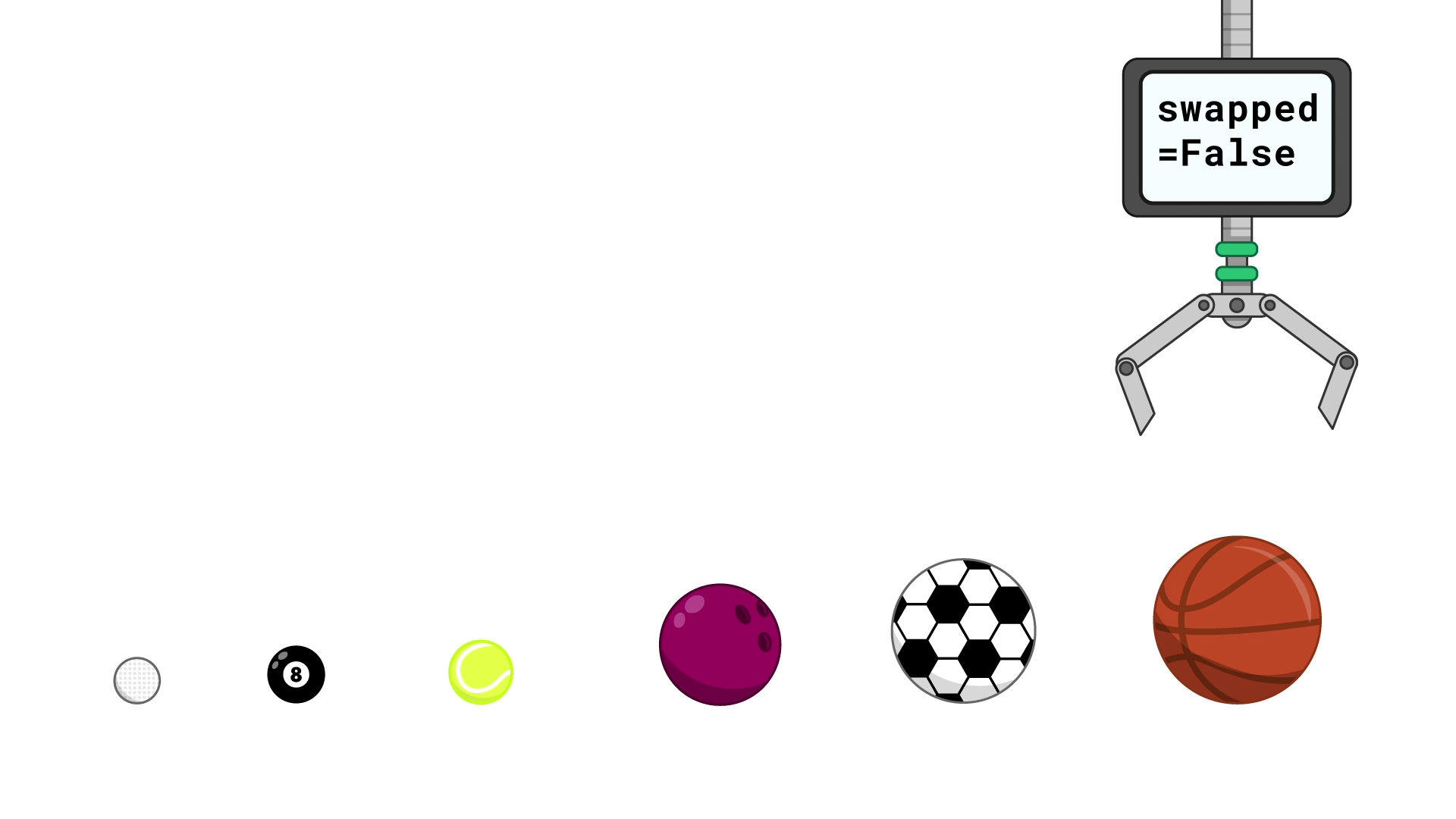What other analogies, scenarios or activities could you use to explain the bubble sort to your learners? Use the comments section to share your thoughts.